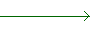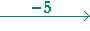Lesson 35

Simultaneous equations:  Section 2

Back to Section 1

Cramer's Rule: The method of determinants

Example 4.   Solve this system of simultaneous equations:

 1) 3x + 4y = 19 2) 2x − y = 9

Solution.   If we add the equations as they are, neither one of the unknowns will cancel.  Now, if the coefficient of y in equation 2) were −4, then the y's would cancel.  Therefore we will expand our strategy as follows:

Make one pair of coefficients negatives of one another -- by multiplying
both sides of an equation by the same number.  Upon adding the equations, that unknown will be eliminated.

To make the coefficients of the y's 4 and −4, we will multiply both sides of equation 2) by 4 :

 1) 3x + 4y = 193x + 4y = 19 2) 2x − y = 98x − 4y = 3611x = 55 x = 5511 x = 5

The 4 over the arrow in equation 2) signifies that both sides of that equation have been multiplied by 4.  Equation 1) has not been changed.

To solve for y, substitute  x = 5  in either one of the original equations.  In equation 1):

 3· 5 + 4y = 19 4y = 19 − 15 4y = 4 y = 1

The solution is (5, 1).

The student should always verify the solution by replacing x and y with (5, 1) in the original equations.

Example 5.   Solve simultaneously:

 1) 3x + 2y = −2 2) 2x + 5y = −5

Solution.   We must make one pair of coefficients negatives of one another.  In this example, we must decide which of the unknowns to eliminate, x or y.  In either case, we will make the new coefficients the Lowest Common Multiple (LCM) of the original coefficients -- but with opposite signs.

Thus, if we eliminate x, then we will make the new coeffients 6 and −6.  (The LCM of 3 and 2 is 6.)  While if we eliminate y, we will make their new coefficients 10 and −10.  (The LCM of 2 and 5 is 10.)

Let us choose to eliminate x:

 1) 3x + 2y = −26x + 4y = −4 2) 2x + 5y = −5−6x − 15y = 15 ________________________________________________________________________ − 11y = 11 y = −1.

Equation 1) has been multiplied by 2.  Equation 2) has been multiplied by −3 -- because we want to make those coefficients  6 and −6, so that on adding, they will cancel.

To solve for x, we will substitute  y = −1  in the original equation 1):

 3x + 2(−1) = −2 3x − 2 = −2 3x = 0 x = 0

The solution is (0, −1).

Problem 3.   Solve simultaneously.

 1) 2x + 3y = 13 2) 5x − y = 7

To make the y's cancel, multiply equation 2) by 3:

 1) 2x + 3y = 132x + 3y = 13 2) 5x − y = 715x − 3y = 21 ________________________________________________________________________ 17x = 34 x = 2

To solve for y:

Substitute  x = 2  in one of the original equations.
In equation 1:

 2· 2 + 3y = 13 4 + 3y = 13 3y = 9 y = 3

The solution is (2, 3).

Problem 4.   Solve simultaneously.

 1) x + 2y = −1 2) 2x − 3y = 5

To make the x's cancel, multiply equation 1) by −2:

 1) x + 2y = −1−2x − 4y = 2 2) 2x − 3y = 52x − 3y = 5 ________________________________________________________________________ − 7y = 7 y = −1

To solve for x:

Substitute  y = −1  in one of the original equations.
In equation 1:

 x + 2(−1) = −1 x − 2 = −1 x = −1 + 2 x = 1

The solution is (1, −1).

We could have eliminated y  by multiplying equation 1) by 3  and equation 2) by 2.

Problem 5.   Solve simultaneously:

 1) 3x − 4y = 1 2) 2x + 3y = 12

To make the y's cancel:

Multiply equation 1) by 3  and equation 2) by 4:

 1) 3x − 4y = 19x − 12y = 3 2) 2x + 3y = 128x + 12y = 48 ________________________________________________________________________ 17x = 51 x = 5117 x = 3

To solve for y:

Substitute  x = 3  in one of the original equations.
In equation 2 (because the sign of y is already positive):

 2· 3 + 3y = 12 6 + 3y = 12 3y = 6 y = 2

The solution is (3, 2).

Problem 6.   Solve simultaneously:

 1) 3x + 2y = −4 2) 2x + 5y = 1

To make the x's cancel:

Multiply equation 1) by 2  and equation 2) by −3:

 1) 3x + 2y = −46x + 4y = −8 2) 2x + 5y = 1−6x − 15y = −3 ________________________________________________________________________ − 11y = −11 y = 1

To solve for x:

Substitute  y = 1  in one of the original equations.
In equation 1:

 3x + 2· 1 = −4 3x + 2 = −4 3x = −4 − 2 3x = −6 x = −2

The solution is (−2, 1).

We could have eliminated y  by multiplying equation 1) by 5  and equation 2) by −2.

Problem 7.   Solve simultaneously:

 1) 5x + 3y = −11 2) 2x + 4y = −10

To make the x's cancel:

Multiply equation 1) by 2  and equation 2) by −5:

 1) 5x + 3y = −1110x + 6y = −22 2) 2x + 4y = −10−10x − 20y = 50 ________________________________________________________________________ − 14y = 28 y = −2

To solve for x:

Substitute  y = −2  in one of the original equations.
In equation 1:

 5x + 3(−2) = −11 5x − 6 = −11 5x = −11 + 6 5x = −5 x = −1

The solution is (−1, −2).

We could have eliminated y  by multiplying equation 1) by 4  and equation 2) by −3.

Cramer's Rule: The method of determinants

A system of two equations in two unknowns has this form:The a's are the coefficients of the x's.  The b's are the coefficients of the y's.  The following is the matrix of those coefficients.The number  a1b2b1a2  is called the determinant of that matrix.

 det= a1b2 − b1a2

Let us denote that determinant by D.

Now consider this matrix in which the c's replace the coefficients of the x's:Then the determinant of that matrix -- which we will call Dx -- is

c1b2b1c2

And consider this matrix in which the c's replace the coeffients of the y's:The determinant of that matrix -- Dy -- is

a1c2c1a2

Cramer's Rule then states the following:

In every system of two equations in two unknowns
in which the determinant D is not 0,

 x = Dx D y = Dy D

Example.   Use Cramer's Rule to solve this system of equations (Problem 7):

 5x + 3y = −11 2x + 4y = −10

Solution.

 D = det= 5· 4 − 3· 2 = 20 − 6 = 14. Dx = det= −11· 4 − 3· −10 = −44 + 30 = −14. Dy = det= 5· −10 − (−11)· 2 = −50 + 22 = −28.

Therefore,

 x = Dx D = −14 14 = −1. y = Dy D = −28 14 = −2.

Problem.   Use Cramer's Rule to solve these simultaneous equations.

 3x − 5y = −31 2x + y = 1
 D = det= 3· 1 − (−5)· 2 = 3 + 10 = 13. Dx = det= −31· 1 − (−5)· 1 = −31 + 5 = −26. Dy = det= 3· 1 − (−31)· 2 = 3 + 62 = 65.

Therefore,

 x = Dx D = −26 13 = −2. y = Dy D = 6513 = 5.

When the determinant D is not 0, we say that the equations are linearly independent. In any system of linearly independent equations, there is one and only one solution.

When the determinant D is 0, then either 1) there is not a unique solution, it is possible to name many; or 2) there is no solution at all. In case 1), the equations are linearly dependent. One of them is simply a multiple of the other. For example,

x + y = 3

2x + 2y = 6.

In case 2), the equations are inconsistent.

x + y = 3

x + y = 4.

Section 3:  Three equations in three unknowns

Back to Section 1Next Lesson:  Word problems that lead to simultaneous equations

Please make a donation to keep TheMathPage online.
Even \$1 will help.